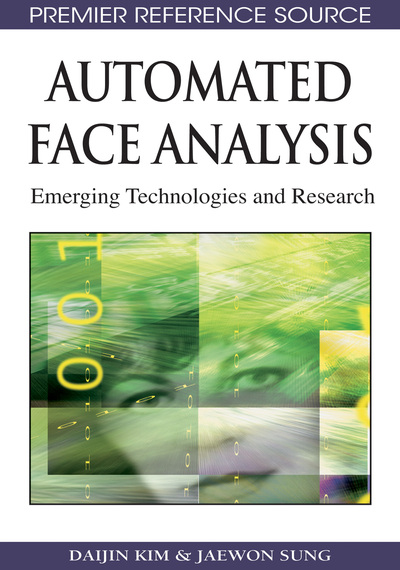# Face Modeling

Daijin Kim (Pohang University of Science & Technology, Korea) and Jaewon Sung (LG Electronics, Korea)
DOI: 10.4018/978-1-60566-216-9.ch003
Available
\$37.50
No Current Special Offers

## Abstract

In the field of computer vision, researchers have proposed many techniques for representation and analysis of the varying shape of objects, such as active contour (Kass et. al., 1998) and deformable template (Yuille et. al., 1989). However, the active contour, which consists of a set of points, is too flexible to limit its deformation to a reasonable amount of variations for a specific object and it does not have the ability to specify a specific shape. The deformable template, which consists of a set of parametric curves, is difficult to represent all the shape deformations of an object due to 3D rotation or self-deformations because the deformations are too complex to be explained by the combination of hand crafted simple parametric curves.
Chapter Preview
Top

## 3.1 Active Shape Models

Cootes et. al. (1995) used the term ASM as the name of the model fitting algorithm in their paper, where the model includes a point distribution model (PDM). The PDM is a kind of deformable shape model that consists of a linear model to explains the shape variation of the training data. This section explains how the PDM can be constrcted from example data and how to find a set of optimal parameters of the PDM for a new input image.

### 3.1.1 Point Distribution Model

In the PDMs, a 2D shape is represented by a set ofvertices, which correspond to the salient points of a non-rigid object. The shape vector s consists of the coordinates of the vertices as

(3.1.1) (3.1.2) whereare the shape parameters.

The mean and shape bases are learned from a set of training images using a statistical analysis technique, principal component analsys (PCA). The standard approach is to apply the PCA to a set of shape vectors that are gathered from the manually landmarked training images. The mean shapeis the mean of gathered shape vectors and the basis vectorsare theeigenvectors corresponding to thelargest eigenvalues.

Usually, the gathered training shape vectors are aligned using the Procrustes analysis (Cootes et. al., 2001d) before the PCA is applied to remove variations due to similarity transformation that scales, rotates, and translates the shape. Thus, the PCA is only concerned with the local shape deformation. Figure 1 illustrates an example of shape bases, where the five shape basestoare displayed over the mean shape.

Because the PDM does not explain the similarity transformation (scaling, rotation, and translation) of the shape, we need 4 more parameters to explain the similarity transformation of the shape when we synthesize a shape using the PDM that coincident with the object in an image. The similarity transformation can be explained by 4 parametersas. (3.1.3)

The PDM is an accurate, specific, and compact deformable shape model. The PDM is an accurate model, which means that it can synthesize all valid shapes. The PDM is a specific model, which means that it excludes all invalid shapes; The invalid shapes can be excluded by simply limiting the range of the shape parameters into a certain range (usually, +/- 3 standard deviations) because the shape parameters are uncorrelated. The PDM is a compact model, which means that it uses the smallest number of parameters that are enough to describe all the shape variations.

## Complete Chapter List

Search this Book:
Reset